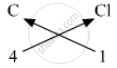SSC (English Medium) Class 8Maharashtra State Board
Share

# Deduce the Molecular Formulae of the Compound Obtained from the Following Pairs of Elements by the Cross Multiplication Method. - SSC (English Medium) Class 8 - Science

ConceptMolecular Formula and Valency

#### Question

Deduce the molecular formulae of the compound obtained from the following pairs of elements by the cross multiplication method.

C (Valency 4) & Cl (Valency 1)

#### Solution

C (Valency 4) & Cl (Valency 1) :
Step 1 : Write the symbols of the radicals.
C                       Cl
Step 2 : Write the valency below the respective radical.
C                        Cl
4                        1
Step 3 : Cross-multiply symbols of radicals with their respective valency.Step 4 : Write down the chemical formula of the compound.
CCl4

Is there an error in this question or solution?

#### APPEARS IN

Balbharati Solution for Balbharati Class 8 Science - General Science (2018 to Current)
Chapter 6: Composition of Matter
Exercise | Q: 8.1 | Page no. 47
Solution Deduce the Molecular Formulae of the Compound Obtained from the Following Pairs of Elements by the Cross Multiplication Method. Concept: Molecular Formula and Valency.
S